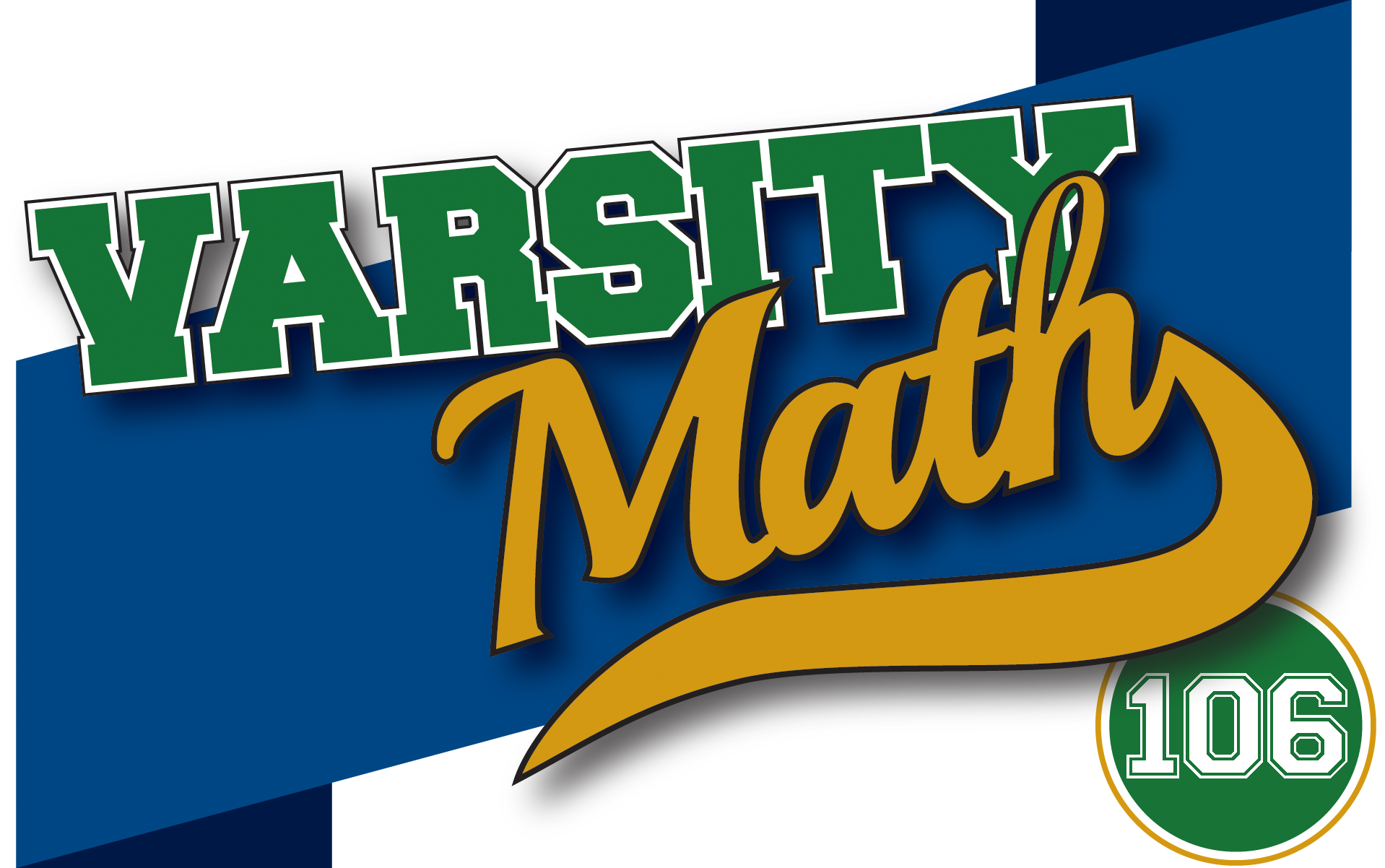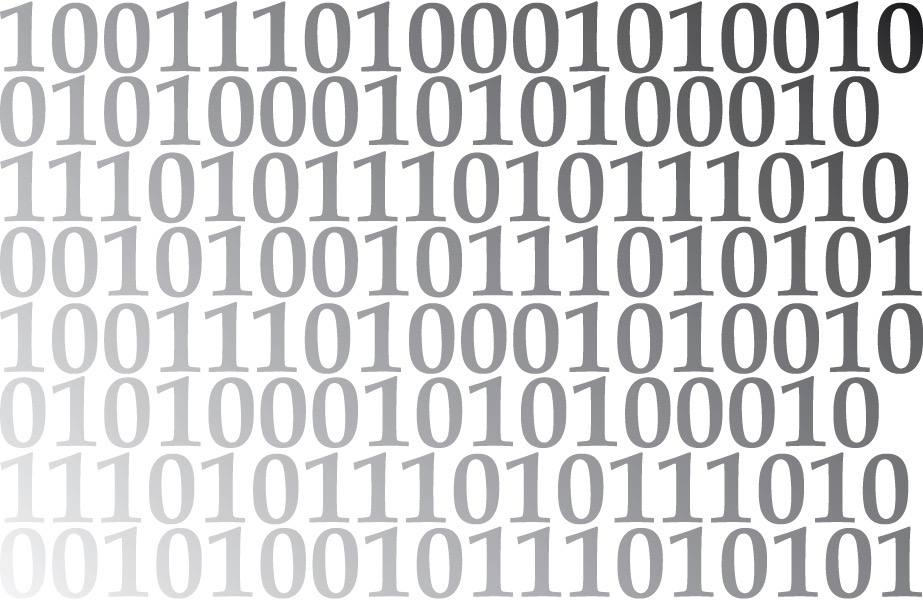## ________________

The team loves puzzles about integers, so today’s problems are right up their alley.

## ________________Define an integer to be simple if it consists exclusively of zeros and ones.

### Simple Multiple

What is the smallest positive simple integer that is a multiple of 14?

### Small and Simple

Find the ten smallest simple integers divisible by 45. Let A be the average of these ten numbers.

What is the value of A + 1?

## Solutions to week 105

In Three Racers, Casey beats Liam by 14.5 meters. The Math for the Ages problem, as worded, led to more than one answer, as several commenters noted. The question should have read: At the math tournament, three volunteers — a woman, her daughter, and her granddaughter — have written to the competition’s founder that their ages multiplied together equal 15,400, and the sum of their ages equals that of the competition’s founder. The founder could not determine their ages. The volunteers are each at least 16 years apart in age. What are the ages of the volunteers and the founder? The answer: The volunteers are 11, 28 and 50, and the founder’s age is 89. We apologize for the omission.

Math for the Ages answer explained: The founder knows his/her own age, but the fact that the founder is unable to determine the ages of the three volunteers means that there must be more than one combination of volunteer ages that add/multiply as described. Once the reader knows that the founder can’t determine the ages of the volunteers, then the reader realizes that the founder’s age must allow for some ambiguity. Only the ages 86, 89, 101, and 102 work in this regard — all have multiple combinations of volunteer ages that add/multiply as described. And then, the reader just needs to determine which of the combinations is the right one, and that’s where the difference in ages of at least 16 years comes in. Only 89 both includes the required ambiguity and allows the volunteer ages to meet the requirement that the ages be at least 16 years apart.

Three Racers answer explained: Assume the time it takes Casey to run 100 meters is T. Rory runs 95 meters in the same amount of time T, which means Rory runs 100 meters in (100/95)*T. Liam runs 90 meters in the same time Rory runs 100 meters, which means Liam runs 100 meters in (100/95)*T*(100/90). To compare the finish times of Casey and Liam, we need to know how far Liam has run in time T, when Casey finishes the 100-meter race. Liam’s speed can be calculated as 100 meters divided by the time it takes to run that 100 meters, or 100/[(100/95)*T*(100/90)], or more simply, (95*90)/(T*100). The distance Liam travels in time T is therefore T*[(95*90)/(T*100)], or (95*90)/100, which is 85.5 meters. Therefore, Casey, who finishes the 100-meter race in time T, finishes 14.5 meters ahead of Liam.

## Recent Weeks

Links to all of the puzzles and solutions are on the Complete Varsity Math page.

Come back next week for answers and more puzzles.